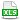HomeTechniques and Tips@RISK Simulation: Numerical ResultsPlacing Iteration Data in Worksheet with RiskData( )

# 6.16. Placing Iteration Data in Worksheet with RiskData( )

Applies to:
@RISK for Excel 4.x–7.x
RISKOptimizer 1.x, 5.x

The manual and the help file say that I can get data from a range of iterations by entering RiskData( ) as an array formula. What does that mean, and can you give an example?

If you want data from all iterations of all inputs and outputs, you can use the Simulation data window (x-subscript-i icon) or select the Simulation Data Excel report.  If you want only selected variables or iterations, use the RiskData( ) worksheet function.

You cannot fill an array with RiskData( ) by typing the formula in one cell and dragging, the way you usually would. Instead, follow this procedure:

1. Select the row or column array where you want to place the input or output value for each iteration.

2. In the formula bar, type your formula, which involves RiskData( ). For instance, to capture the first 100 iterations of the input called The_Input, type

=RiskData("The_Input",1)

To capture iterations 151 through 250 of cell A4, type

=RiskData(A4,151)

An optional third argument to RiskData( )lets you specify the simulation number, if you're running RISKOptimizer or multiple simulations in @RISK.

3. Instead of Enter, press Ctrl-Shift-Enter to create an array formula for this array. Though Excel puts curly braces { } around the formula, you can't create an array formula by typing curly braces yourself.

4. If you haven't run a simulation, you'll see lots of #N/A appear in the array. These will change to numbers when you run your simulation. To see this happen, open the attached example and run a simulation.

See also: In @RISK 6.0 and later, if you just have an occasional need you can get all the iterations for one input or output in the Browse Results window. See All Iterations of One Input or Output.

Last edited: 2015-06-30

•RiskDataArray.xls